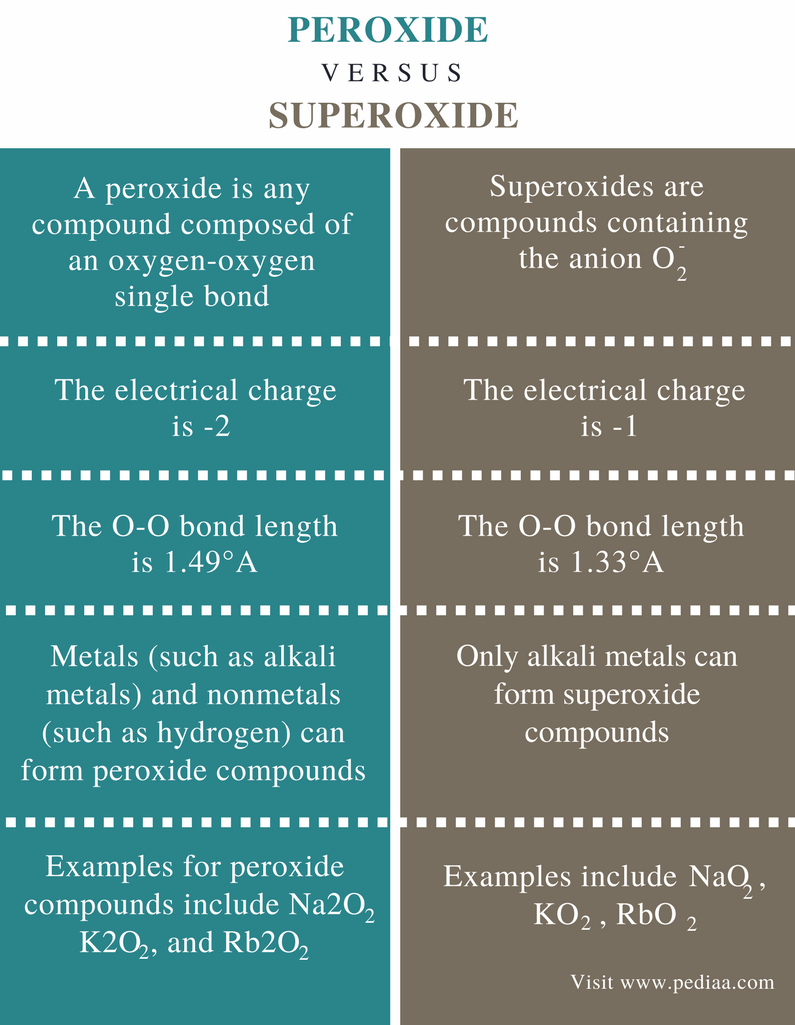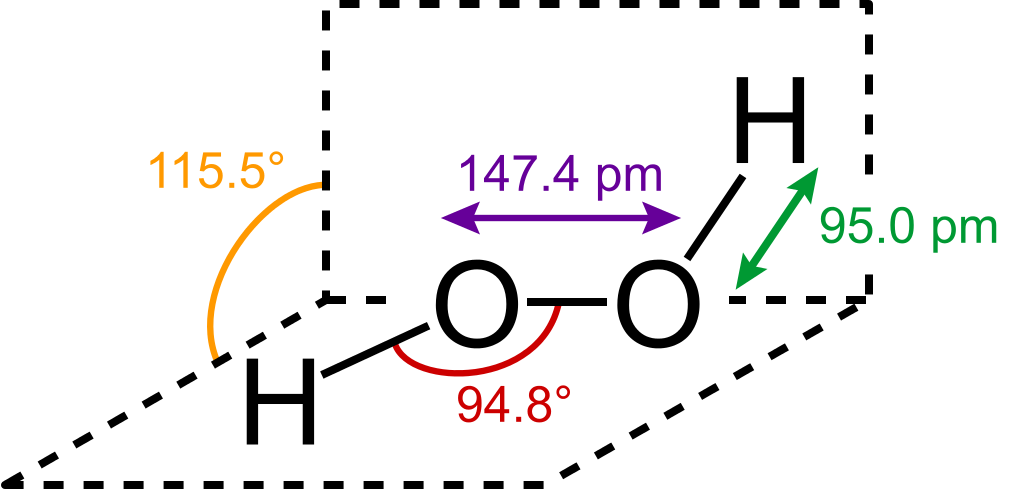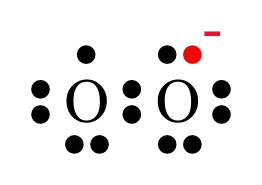# Difference Between Peroxide and Superoxide

## Main Difference – Peroxide vs Superoxide

An oxide is any chemical compound that contains one or more oxygen atoms. Oxides can be oxides containing oxide anions (O2-), peroxides containing peroxide anions (O) or superoxides containing superoxide anion (O2). A peroxide is any compound that is composed of an oxygen-oxygen single bond. This can be either in the form of an anion or in between other atoms of the molecule. Hydrogen peroxide is the simplest peroxide that can be found. Superoxide is composed of highly reactive oxygen atoms. Superoxides are formed only by alkali metals (group 1 elements). The main difference between peroxide and superoxide is that the oxidation state of oxygen in peroxide is -1 whereas the oxidation state of oxygen in superoxide is -1/2.

### Key Areas Covered

1. What is Peroxide
– Definition, Structure, Examples
2. What is Superoxide
– Definition, Structure, Examples
3. What is the Difference Between Peroxide and Superoxide
– Comparison of Key Differences

Key Terms: Alkali Metal, Alkaline Earth Metals, Anion, Bond Length, Metals, Nonmetals, Oxide, Peroxide, Peroxide Anion, Peroxo, Superoxide## What is Peroxide

A peroxide is any compound composed of an oxygen-oxygen single bond. Therefore, the main feature to identify a peroxide compound is the presence of this O-O covalent bond. Sometimes, this bond can be observed as an anion in an ionic compound. Then it is given in symbols as O2-2. This is called the peroxide anion. When the O-O bond is found in a covalent compound, it is called a peroxo group or peroxide group.Figure 1: H2O2 is the Simplest Peroxide

In the peroxide group, the oxidation state of one oxygen atom is -1. Normally oxygen prefers to show the oxidation state 0 or -2. But since two oxygen atoms are bonded to each other, one oxygen atom gets a -1 oxidation state. In the peroxide anion, one oxygen atom has -1 electrical charge since the overall charge of the anion is -2.

Ionic peroxides are composed of a peroxide anion bonded to alkali metal ions or alkaline earth metal ions as the cation. Some examples are sodium peroxide (Na2O2), potassium peroxide (K2O2), Magnesium peroxide (MgO), etc. Covalent peroxide compounds are composed of O-O single bond directly bonded to other atoms in the molecule; for example, hydrogen peroxide (H2O2) and peroxymonosulfuric acid (H2SO5).

Peroxides can be found in biological systems and in nature. For example, some enzymes in our cells use peroxides to catalyze certain reactions. Some plant species use peroxide compounds as signaling chemicals. Peroxides are used in laboratory scale applications as well. It is very useful in organic chemistry in order to obtain the anti-Markovnikov product from an organic reaction.

## What is Superoxide

Superoxides are compounds containing the anion O2. In the superoxide group, the oxidation state of one oxygen atom is -1/2. Normally oxygen prefers to show the oxidation state 0 or -2. But since there are two oxygen atoms are bonded to each other, one oxygen atom gets a -1/2 oxidation state. In the superoxide anion, one oxygen atom has -1/2 electrical charge since the overall charge of the anion is -1.Figure 2: Chemical Structure of Superoxide Anion

This anion is also composed of O-O single bond. The superoxide anion is highly reactive because the -1/2 oxidation state is not stable. Superoxide anion is considered as a free radical that exhibit paramagnetism. That is due to the presence of an unpaired electron on one oxygen atom (as shown in the above image).

The bond distance for O-O bond in superoxide anion is about 1.33oA. Only alkali metals tend to form superoxide compounds. They form the superoxide compound via direct reaction with O2. The superoxides of alkali metals include NaO2, KO2, RbO2 and CsO2. Here, the metal has +1 electrical charge. Correspondingly, the anion should have -1 electrical charge in order to neutralize the compound.

When these superoxide compounds are dissolved in water, the system undergoes disproportionation rapidly. Disproportionation is a redox reaction in which a compound of intermediate oxidation state converts to two different compounds. Here, superoxide anion and water react to form O2 and OH ion.

4O2   +   2H2O   →   3O2   +   4OH

This reaction is why potassium superoxide is used as an oxygen source in chemical oxygen generators in space shuttles and submarines.

## Difference Between Peroxide and Superoxide

### Definition

Peroxide: A peroxide is any compound composed of an oxygen-oxygen single bond.

Superoxide: Superoxides are compounds containing the anion O2.

### Chemical Formula

Peroxide: The chemical formula of peroxide anion is O2-2.

Superoxide: The chemical formula of superoxide anion is O2.

### Electrical Charge

Peroxide: The electrical charge of peroxide ion is -2.

Superoxide: The electrical charge of superoxide ion is -1.

### Bond Length

Peroxide: The O-O bond length in peroxide ion is 1.49°A.

Superoxide: The O-O bond length in superoxide ion is 1.33°A.

### Nature

Peroxide: Metals (such as alkali metals) and nonmetals (such as hydrogen) can form peroxide compounds.

Superoxide: Only alkali metals can form superoxide compounds.

### Examples

Peroxide: Examples for peroxide compounds include Na2O2, K2O2, Rb2O2 and Cs2O2

Superoxide: Examples for superoxide compounds include NaO2, KO2, RbO2 and CsO2.

### Conclusion

Peroxide and superoxide are oxides containing oxygen atoms. The main difference between peroxide and superoxide is that the oxidation state of oxygen in peroxide is -1 whereas the oxidation state of oxygen in superoxide is -1/2.

##### Reference:

1.  “12.4: Peroxides and Superoxides.” Chemistry LibreTexts, Libretexts, 21 July 2016, Available here.
2. “Superoxide.” Wikipedia, Wikimedia Foundation, 15 Dec. 2017, Avialable here.

##### Image Courtesy:

1. “H2O2 gas structure” By SVG: Sassospicco; Raster: Walkerma – File:H2O2 structure.png (GFDL) via Commons Wikimedia
2. “Superoxide” (Public Domain) via Commons Wikimedia